### Open Methods: Newton Raphson Method

### The Method

The Newton-Raphson method is one of the most used methods of all root-finding methods. The reason for its success is that it converges very fast in most cases. In addition, it can be extended quite easily to multi-variable equations. To find the root of the equation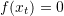, the Newton-Raphson method depends on the Taylor Series Expansion of the function around the estimate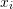to find a better estimate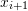: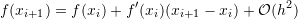whereis the estimate of the root after iteration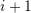andis the estimate at iteration. Assuming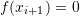and rearranging: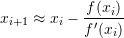The procedure is as follows. Setting an initial guess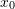, tolerance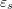, and maximum number of iterations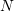:
At iteration, calculate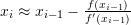and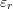. If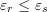or if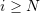, stop the procedure. Otherwise repeat.
Note: unlike the previous methods, the Newton-Raphson method relies on calculating the first derivative of the function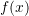. This makes the procedure very fast, however, it has two disadvantages. The first is that this procedure doesn’t work if the functionis not differentiable. Second, the inverse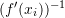can be slow to calculate when dealing with multi-variable equations.

##### Example

As an example, let’s consider the function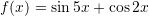. The derivative of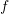is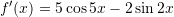. Setting the maximum number of iterations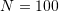,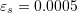,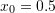, the following is the Microsoft Excel table produced: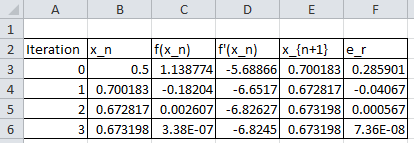Mathematica has a built-in algorithm for the Newton-Raphson method. The function “FindRoot[lhs==rhs,{x,x0}]” applies the Newton-Raphson method with the initial guess being “x0”. The following is a screenshot of the input and output of the built-in function evaluating the roots based on three initial guesses.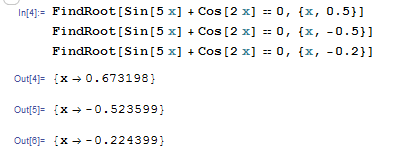Alternatively, a Mathematica code can be written to implement the Newton-Raphson method with the following output for three different initial guesses: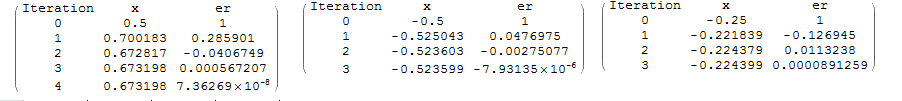View Mathematica Code
Clear[x]
f[x_] := Sin[5 x] + Cos[2 x]
fp = D[f[x], x]
xtable = {-0.25};
er = {1};
es = 0.0005;
MaxIter = 100;
i = 1;
While[And[i <= MaxIter, Abs[er[[i]]] > es], xnew = xtable[[i]] - (f[xtable[[i]]]/fp /. x -> xtable[[i]]);  xtable = Append[xtable, xnew]; ernew = (xnew - xtable[[i]])/xnew;  er = Append[er, ernew]; i++];
T = Length[xtable];
SolutionTable = Table[{i - 1, xtable[[i]], er[[i]]}, {i, 1, T}];
SolutionTable1 = {"Iteration", "x", "er"};
T = Prepend[SolutionTable, SolutionTable1];
T // MatrixForm

View Python Code
import sympy as sp
import pandas as pd

def f(x): return sp.sin(5*x) + sp.cos(2*x)
x = sp.symbols('x')
fp = f(x).diff(x)
xtable = [-0.25]
er = 
es = 0.0005
MaxIter = 100
i = 0
while i <= MaxIter and abs(er[i]) > es:
xnew = xtable[i] - (f(xtable[i])/fp.subs(x,xtable[i]))
xtable.append(xnew)
ernew = (xnew - xtable[i])/xnew
er.append(ernew)
i+=1
SolutionTable = [[i, xtable[i], er[i]] for i in range(len(xtable))];
sol = pd.DataFrame(SolutionTable,columns=["Iteration", "x", "er"])
display(sol)

# Scipy's root function
import numpy as np
from scipy.optimize import root
def f(x):
return np.sin(5*x) + np.cos(2*x)
sol = root(f,)
print("Scipy's Root: ",sol.x)


The following MATLAB code runs the Newton-Raphson method to find the root of a functionwith derivative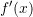and initial guess. The value of the estimate and approximate relative error at each iteration is displayed in the command window. Additionally, two plots are produced to visualize how the iterations and the errors progress. You need to pass the function f(x) and its derivative df(x) to the newton-raphson method along with the initial guess as newtonraphson(@(x) f(x), @(x) df(x), x0). For example, try newtonraphson(@(x) sin(5.*x)+cos(2.*x), @(x) 5.*cos(5.*x)-2.*sin(2.*x),0.4)

### Analysis of Convergence of the Newton-Raphson Method

The error in the Newton-Raphson Method can be roughly estimated as follows. The estimateis related to the previous estimateusing the equation: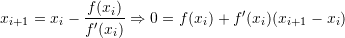Additionally, using Taylor’s theorem, and if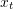is the true root withwe have: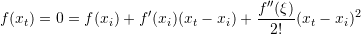for somein the interval betweenand. Subtracting the above two equations yields: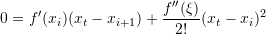Since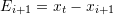and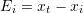then:

(1)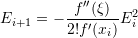If the method is converging, we have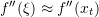and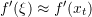therefore: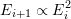Therefore, the error is squared after each iteration, i.e., the number of correct decimal places approximately doubles with each iteration. This behaviour is called quadratic convergence. Look at the tables in the Newton-Raphson example above and compare the relative error after each step!

The following tool can be used to visualize how the Newton-Raphson method works. Using the slope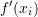, the difference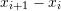can be calculated and thus, the value of the new estimatecan be computed accordingly. Use the slider to see how fast the method converges to the true solution using,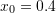, and solving for the root of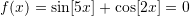.

View Mathematica code
Manipulate[
f[x_] := Sin[5 x] + Cos[2 x];
fp = D[f[x], x];
xtable = {0.4};
er = {1};
es = 0.0005;
MaxIter = 100;
i = 1;
While[And[i <= MaxIter, Abs[er[[i]]] > es], xnew = xtable[[i]] - (f[xtable[[i]]]/fp /. x -> xtable[[i]]); xtable = Append[xtable, xnew]; ernew = (xnew - xtable[[i]])/xnew; er = Append[er, ernew]; i++];
T = Length[xtable];
SolutionTable = Table[{i - 1, xtable[[i]], er[[i]]}, {i, 1, T}];
SolutionTable1 = {"Iteration", "x", "er"};
T = Prepend[SolutionTable, SolutionTable1];
LineTable1 = Table[{{T[[i, 2]], 0}, {T[[i, 2]], f[T[[i, 2]]]}, {T[[i + 1, 2]],0}}, {i, 2, n - 1}];
Grid[{{Plot[f[x], {x, 0, 1}, PlotLegends -> {"f(x)"}, ImageSize -> Medium,  Epilog -> {Dashed, Line[LineTable1]}]}, {Row[{"Iteration=", n - 2, "    x_n=", T[[n, 2]], "   f(x_n)=", f[T[[n, 2]]]}]}}], {n, 2, 7, 1}]

View Python Code
import numpy as np
import sympy as sp
import matplotlib.pyplot as plt
from ipywidgets.widgets import interact

def f(x): return sp.sin(5*x) + sp.cos(2*x)
x = sp.symbols('x')
fp = f(x).diff(x)

@interact(n=(2,7,1))
def update(n=2):
xtable = [0.4]
er = 
es = 0.0005
MaxIter = 100
i = 0
while i <= MaxIter and abs(er[i]) > es:
xnew = xtable[i] - (f(xtable[i])/fp.subs(x,xtable[i]))
xtable.append(xnew)
ernew = (xnew - xtable[i])/xnew
er.append(ernew)
i+=1
SolutionTable = [[i - 1, xtable[i], er[i]] for i in range(len(xtable))]
LineTable = [[],[]]
for i in range(n-2):
LineTable.extend([SolutionTable[i], SolutionTable[i], SolutionTable[i + 1]])
LineTable.extend([0, f(SolutionTable[i]), 0])

x_val = np.arange(0,1.1,0.03)
y_val = np.sin(5*x_val) + np.cos(2*x_val)
plt.plot(x_val,y_val, label="f(x)")
plt.plot(LineTable,LineTable,'k--')
plt.legend(); plt.grid(); plt.show()
print("Iteration =",n-2," x_n =",round(SolutionTable[n-2],5)," f(x_n) =",f(SolutionTable[n-2]))


Depending on the shape of the function and the initial guess, the Newton-Raphson method can get stuck around the locations of oscillations of the function. The tool below visualizes the algorithm when trying to find the root of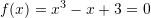with an initial guess of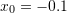. It takes 33 iterations before reaching convergence.

Depending on the shape of the function and the initial guess, the Newton-Raphson method can get stuck in a loop. The tool below visualizes the algorithm when trying to find the root ofwith an initial guess of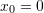. The algorithm goes into an infinite loop.

In general, an initial guess that is close enough to the true root will guarantee quick convergence. The tool below visualizes the algorithm when trying to find the root ofwith an initial guess of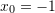. The algorithm quickly converges to the desired root.

The following is the Mathematica code used to generate one of the tools above:

View Mathematica Code
Manipulate[
f[x_] := x^3 - x + 3;
fp = D[f[x], x];
xtable = {-0.1};
er = {1.};
es = 0.0005;
MaxIter = 100;
i = 1;
While[And[i <= MaxIter, Abs[er[[i]]] > es], xnew = xtable[[i]] - (f[xtable[[i]]]/fp /. x -> xtable[[i]]); xtable = Append[xtable, xnew]; ernew = (xnew - xtable[[i]])/xnew; er = Append[er, ernew]; i++];
T = Length[xtable];
SolutionTable = Table[{i - 1, xtable[[i]], er[[i]]}, {i, 1, T}];
SolutionTable1 = {"Iteration", "x", "er"};
T = Prepend[SolutionTable, SolutionTable1];
LineTable1 =   Table[{{T[[i, 2]], 0}, {T[[i, 2]], f[T[[i, 2]]]}, {T[[i + 1, 2]], 0}}, {i, 2, n - 1}];
Grid[{{Plot[f[x], {x, -3, 2}, PlotLegends -> {"f(x)"}, ImageSize -> Medium, Epilog -> {Dashed, Line[LineTable1]}]}, {Row[{"Iteration=", n - 2, "    x_n=", T[[n, 2]], "   f(x_n)=", f[T[[n, 2]]]}]}}] , {n, 2, 33, 1}]

View Python Code
import numpy as np
import sympy as sp
import matplotlib.pyplot as plt
from ipywidgets.widgets import interact

def f(x): return x**3 - x + 3
x = sp.symbols('x')
fp = f(x).diff(x)

@interact(n=(2,33,1))
def update(n=2):
xtable = [-0.1]
er = 
es = 0.0005
MaxIter = 100
i = 0
while i <= MaxIter and abs(er[i]) > es:
xnew = xtable[i] - (f(xtable[i])/fp.subs(x,xtable[i]))
xtable.append(xnew)
ernew = (xnew - xtable[i])/xnew
er.append(ernew)
i+=1
SolutionTable = [[i - 1, xtable[i], er[i]] for i in range(len(xtable))]
LineTable = [[],[]]
for i in range(n-2):
LineTable.extend([SolutionTable[i], SolutionTable[i], SolutionTable[i + 1]])
LineTable.extend([0, f(SolutionTable[i]), 0])

x_val = np.arange(-3,2.2,0.03)
y_val = x_val**3 - x_val + 3
plt.plot(x_val,y_val, label="f(x)")
plt.plot(LineTable,LineTable,'k--')
plt.xlim(-3,2.2); plt.ylim(-25,10)
plt.legend(); plt.grid(); plt.show()
print("Iteration =",n-2," x_n =",round(SolutionTable[n-2],5)," f(x_n) =",round(f(SolutionTable[n-2]),5))


### Lecture video

1.Lei Zhang says:
1.Samer Adeeb says:
2.mary says: